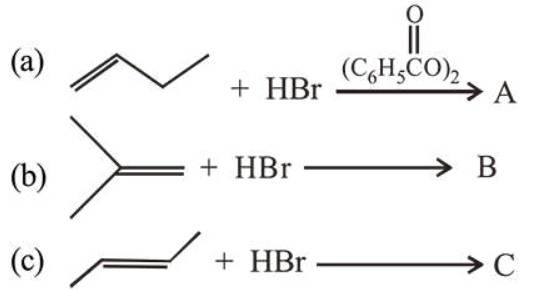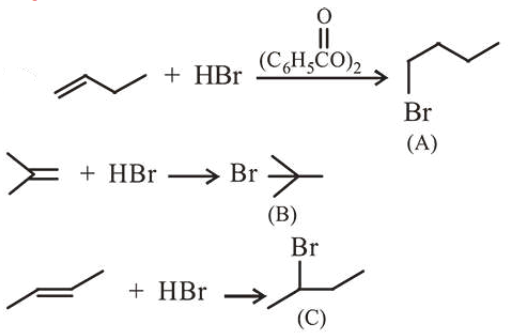# The increasing order of the boiling point of the major products A,Question:

The increasing order of the boiling point of the major products $\mathrm{A}, \mathrm{B}$ and $\mathrm{C}$ of the following reactions will be:1. $\mathrm{B}<\mathrm{C}<\mathrm{A}$

2. $\mathrm{C}<\mathrm{A}<\mathrm{B}$

3. $A 4.$\mathrm{A}<\mathrm{C}<\mathrm{B}\$

Correct Option: 1

Solution:The boiling points of isomeric haloalkanes decrease with increase in branching.

So order of B.P. is A > C > B.